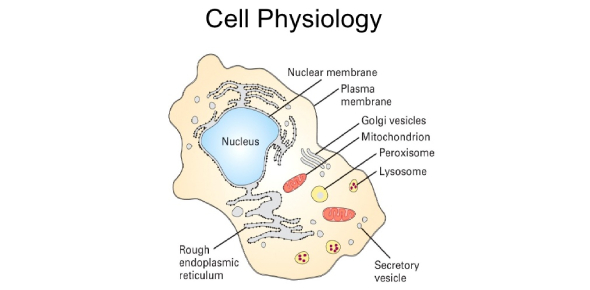# Cell Physiology Chapter 3 Test

26 Questions | Total Attempts: 5292SettingsIn the scientific study of biology, there is a sub-study called cell physiology wherein we monitor the numerous activities that take place within animal cells, plant cells and microorganisms in order to keep them alive. Do you have a good idea of what goes on under the hood of the cell?

• 1.
There are two types of transport through cells: one requires energy and the other does not
• A.

True

• B.

False

• 2.
Hypotonic solutions are so named because their solute content is lower than the solution they are being compared to.
• A.

True

• B.

False

• 3.
Diffusion is important in the study of physiology because it is how most substances enter and exit cells.
• A.

True

• B.

False

• 4.
The distal end of a bone would be the farthest end from a reference point.
• A.

True

• B.

False

• 5.
A solution that is more dilute than the inside of a cell would cause that cell to shrink in size.
• A.

True

• B.

False

• 6.
The dorsal fin of a shark would be visible from the surface of the water.
• A.

True

• B.

False

• 7.
If a substance is more concentrated inside a cell than it is outside, the only way to get more of it into the cell is by active transport.
• A.

True

• B.

False

• 8.
A cell that suddenly stopped producing energy could still carry out diffusion.
• A.

True

• B.

False

• 9.
Animal cells have membranes which allow the passage of selective substances.
• A.

True

• B.

False

• 10.
The collarbone is superior to the pelvic bone.
• A.

True

• B.

False

• 11.
The regulation of blood pressure is a good example of homeostasis.
• A.

True

• B.

False

• 12.
Diffusion is a process where a substance moves from an area of low concentration to an area of high concentration.
• A.

True

• B.

False

• 13.
Oxygen and carbon dioxide can cross the membrane through diffusion.
• A.

True

• B.

False

• 14.
The process of osmosis requires that the cell expend energy
• A.

True

• B.

False

• 15.
When a cube of sugar is placed in water it spreads by the process of active transport
• A.

True

• B.

False

• 16.
Selectively permeable membranes allow only certain substances to pass through them.
• A.

True

• B.

False

• 17.
The sodium/potassium pump moves sodium and potassium against the concentration gradient.
• A.

True

• B.

False

• 18.
The shoulder blade of a dog would be posterior to the hind legs.
• A.

True

• B.

False

• 19.
Substances outside the membrane are called:
• A.

Intracellular material

• B.

Intercellular material

• C.

Extracellular material

• D.

Both A & B

• E.

None of the above

• 20.
The movement of molecules in response to a concentration gradient:
• A.

Aerobic respiration

• B.

Photosynthesis

• C.

Mitosis

• D.

Diffusion

• 21.
Osmosis:
• A.

Involves the diffusion of solute particles

• B.

Can cause a cell to shrink when placed in a hypotonic solution

• C.

Occurs through a non-permeable membrane

• D.

Causes a cell to burst when placed in a hypotonic solution

• 22.
If salt solution A is 3 times as concentrated as salt solution B this means:
• A.

Solution A has 3 times as much salt as solution B

• B.

Solution A has 3 times as much water as solution B

• C.

Solution B has 3 times as much salt as solution A

• D.

Solution A is equal to solution B

• 23.
Solutions injected into the circulatory system must be _____________ in order to prevent damage to cells
• A.

Hypotonic

• B.

Hypertonic

• C.

Isotonic

• D.

Both A & B

• E.

None of the above

• 24.
Carbon dioxide and ___________ are waste products of cellular respiration.
• A.

Water

• B.

Glucose

• C.

Sodium

• D.

Energy

• E.

None of the above

• 25.
Anterior refers to a body part that is toward the:
• A.

Rear

• B.

Bottom

• C.Back to top